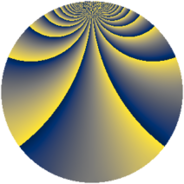# Properties

 Label 882.2.bbLevel $882$ Weight $2$ Character orbit 882.bb Rep. character $\chi_{882}(25,\cdot)$ Character field $\Q(\zeta_{21})$ Dimension $672$ Newform subspaces $2$ Sturm bound $336$ Trace bound $2$

# Related objects

## Defining parameters

 Level: $$N$$ $$=$$ $$882 = 2 \cdot 3^{2} \cdot 7^{2}$$ Weight: $$k$$ $$=$$ $$2$$ Character orbit: $$[\chi]$$ $$=$$ 882.bb (of order $$21$$ and degree $$12$$) Character conductor: $$\operatorname{cond}(\chi)$$ $$=$$ $$441$$ Character field: $$\Q(\zeta_{21})$$ Newform subspaces: $$2$$ Sturm bound: $$336$$ Trace bound: $$2$$ Distinguishing $$T_p$$: $$5$$

## Dimensions

The following table gives the dimensions of various subspaces of $$M_{2}(882, [\chi])$$.

Total New Old
Modular forms 2064 672 1392
Cusp forms 1968 672 1296
Eisenstein series 96 0 96

## Trace form

 $$672q - 112q^{4} + 4q^{5} - 10q^{6} + 2q^{7} - 10q^{9} + O(q^{10})$$ $$672q - 112q^{4} + 4q^{5} - 10q^{6} + 2q^{7} - 10q^{9} - 2q^{13} - 2q^{14} - 6q^{15} - 112q^{16} + 14q^{17} + 8q^{18} + 4q^{19} + 4q^{20} + 20q^{21} - 78q^{23} + 4q^{24} + 56q^{25} + 16q^{26} + 84q^{27} + 2q^{28} - 26q^{29} - 8q^{30} + 4q^{31} + 40q^{33} + 14q^{35} - 10q^{36} + 26q^{37} + 12q^{38} - 16q^{39} + 6q^{41} - 86q^{42} - 2q^{43} + 214q^{45} - 78q^{46} + 30q^{47} - 4q^{49} + 8q^{50} + 6q^{51} + 26q^{52} - 156q^{53} - 14q^{54} - 30q^{55} - 2q^{56} - 48q^{57} + 78q^{58} - 44q^{59} - 6q^{60} - 82q^{61} - 44q^{62} - 24q^{63} - 112q^{64} - 28q^{65} + 16q^{66} + 28q^{67} - 42q^{68} - 66q^{69} + 18q^{70} + 14q^{71} + 8q^{72} + 28q^{73} + 6q^{74} - 34q^{75} + 4q^{76} - 50q^{77} - 8q^{78} + 16q^{79} - 24q^{80} - 46q^{81} + 376q^{83} - 22q^{84} - 4q^{86} - 60q^{87} + 106q^{89} + 82q^{90} + 16q^{91} + 6q^{92} + 76q^{93} + 24q^{94} + 52q^{95} + 4q^{96} - 2q^{97} - 32q^{98} - 190q^{99} + O(q^{100})$$

## Decomposition of $$S_{2}^{\mathrm{new}}(882, [\chi])$$ into newform subspaces

Label Dim. $$A$$ Field CM Traces $q$-expansion
$$a_2$$ $$a_3$$ $$a_5$$ $$a_7$$
882.2.bb.a $$336$$ $$7.043$$ None $$-56$$ $$-5$$ $$2$$ $$0$$
882.2.bb.b $$336$$ $$7.043$$ None $$56$$ $$5$$ $$2$$ $$2$$

## Decomposition of $$S_{2}^{\mathrm{old}}(882, [\chi])$$ into lower level spaces

$$S_{2}^{\mathrm{old}}(882, [\chi]) \cong$$ $$S_{2}^{\mathrm{new}}(441, [\chi])$$$$^{\oplus 2}$$